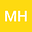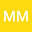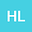loading page

Global weak solution to a generic reaction-diffusion nonlinear parabolic system
•••Matallah HanaLaboratory of Applied Mathematics and History and Didactics of Mathematiccs (LAMAHIS), University of 20 August 1955, skikda, Algeria
Author ProfileMESSAOUD MAOUNI
Laboratory of Applied Mathematics and History and Didactics of Mathematiccs (LAMAHIS), University of 20 August 1955, skikda, Algeria
Author ProfileHakim Lekhal
Laboratory of Applied Mathematics and History and Didactics of Mathematiccs (LAMAHIS), University of 20 August 1955, skikda, Algeria
Author Profile## Abstract

We consider a new generic reaction-diffusion system, given as the following form: ∂u/∂t - div(g(│(∇u_σ)│)∇u)=f(t,x,u,v,∇v), in Q_T ∂v/∂t - d_v Δv=p(t,x,u,v,∇u), in Q_T u(0,.)=u_0, v(0,.)=v_0, in Ω (1) ∂u/∂η=0, ∂v/∂η=0, in ∑_T. Where Ω=]0,1[?×]0,1[, Q_T =]0,T [? and T =]0,T [?, (T > 0), η is an outward normal to domain Ω and u_0, v_0 is the image to be processed, x ∈Ω, σ >0, ∇u_σ= u∗ ∇G_σ and G_σ= 1/√2πσ exp(-│x│^2/4σ). In this study we are going to proof that there is a global weak solution to the ptoblem (1), we truncate the system and show that it can be solved by using Schauder fixed point theorem in Banach spaces. Finally by making some estimations, we prove that the solution of the truncated system converge to the solution of the problem.

#### Peer review status:UNDER REVIEW

23 Feb 2021Submitted to Mathematical Methods in the Applied Sciences
24 Feb 2021Assigned to Editor
24 Feb 2021Submission Checks Completed
03 Mar 2021Reviewer(s) Assigned